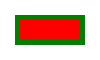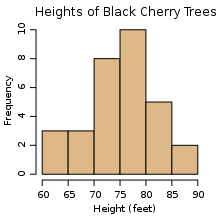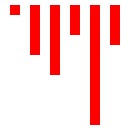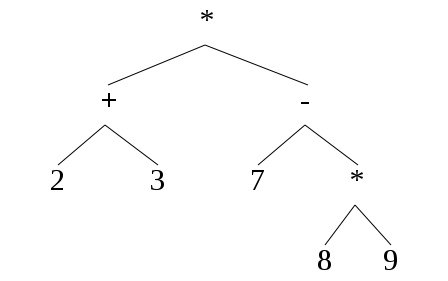aaron maxwell

# SVG, Markup Languages and the Chomsky Hierarchy

Software development sometimes seems divorced from the theory of computer science. Certainly being good with math does not automatically make one a great coder, and vice versa; some different skills and personality traits are required.

Deep down, however, there are certainly places where the two worlds intersect. Some friends and myself came across one recently, at the intersection of two roads: gritty, practical XML markup languages, and the Chomsky Language hierarchy.

SVG is such a markup language. It's an XML specification of 2D vector graphic images, which can be rendered directly or converted to other image file formats.svg source
```<?xml version="1.0" standalone="no"?>
<!DOCTYPE svg PUBLIC "-//W3C//DTD SVG 1.1//EN"
"http://www.w3.org/Graphics/SVG/1.1/DTD/svg11.dtd">
<svg width="100" height="60"
xmlns="http://www.w3.org/2000/svg">
<rect fill="green" x="15" y="15" width="70" height="30"/>
<rect fill="red" x="20" y="20" width="60" height="20"/>
</svg>
```

SVG provides a rich set of primitives and high level features for describing two-dimensional graphics, including operations for styling, object grouping and transformations, and for incorporating text and bitmap images. In addition, it is an open web standard. It's an interesting file format for what it does.

Let's switch topics a moment to the language hierarchy. In the mid-20th century, Noam Chomsky famously outlined a ranking of formal languages based on their expressive power. Here, "formal" means "defineable using the tools of computer science and mathematics." In the end, every general-purpose and specialized programming language is formal in this sense, even if the details of creating the formal description can get messy.

Chomsky's original hierarchy had at least four distinctions, and several more refined distinctions usefully exist. Here, we are concerned with two of those categories: regular languages and context-free languages.

A language, in this context, is just a set of strings. That's all. It can be an infinite set, and in fact, all useful programming languages I know of are infinite. Imagine all possible valid Java programs. That is a set of strings, and hence a language. Now imagine all possible regular expressions. That, too, is a set of strings, and a language. (The word "language" is overloaded a bit, which could confuse us if we allow it. We'll try to dodge that.)

A language can be categorized depending on its expressive power. Two such categories are called "regular" and "context-free". The definitions are quite precise: a language is regular if there exists some finite state machine that accepts all strings in the language and rejects all strings outside of it. And a language is context-free if there exists some context-free grammar that accepts all strings in that language, and rejects those outside of it.

You may be familiar with both finite state machines (FSMs) and context free grammars (CFGs). They are simply mathematical constructs that can be used to define sets of strings. In case you don't recall or never learned, an important fact is that not all languages can be described by a FSM. In other words, some languages are regular, and some are not. Similarly, some languages are context-free (can be described by a CFG), and are not. However, all regular languages are also context-free languages, but not vice versa.

In other words, the set of context-free languages is a strict superset of the set of all regular languages. For this reason, we say that context-free languages have more expressive power than regular languages.

Now, how does this relate to markup languages? Well, XML, and some XML markup languages like SVG and XHTML, are all context free. You cannot create a finite state machine that will correctly discriminate all possible XML (or SVG or XHTML) documents.

However, every context-free language has subsets that are regular. Sometimes this is useful. Let's start with a contrived example: minimal HTML documents that just contain lists. Consider exhibit A:

```<html>
<body>
<ul>
<li>Hello</li>
<li>Handsome</li>
<li>Programmer</li>
</ul>
<ul>
<li>Another</li>
<li>List</li>
</ul>
</body>
</html>
```

This specimin - a multi-line string - is from a language that is a limited subset of XHTML. Namely, those whose body contains only unordered lists, and whose list items contain simple text. It is a regular language; you can craft a FSM that accepts only this language. Now, you may or may not be conversant in building state machines. Regardless, we can just use regular expressions instead: as theorems in any thorough automata textbook will show, if and only if a language is regular, there exists some regular expression that accepts it (and rejects strings not in the language). So one regular expression for Exhibit A is:

```"<html><body>" ("<ul>" ("<li>" text "</li>")* "</ul>")* "</body></html>"
```

This regular expression will only accept these simple-list HTML documents. Well, fine. But what if you need to work with documents like this:

```<html>
<body>
<ul>
<li>Programmers</li>
<li>are</li>
<li>
<ul>
<li>Handsome</li>
<li>Charming</li>
<li>Happy</li>
</ul>
</li>
</ul>
<ul>
<li>Random</li>
<li>Words</li>
</ul>
</body>
</html>
```

Here, there is a nested list, which is definitely not accepted by the regular expression above. Can you write a regular expression that will? Yes, but it would be a little messy, and that's if you limit it to a single level of nesting. Nest deeper, and the regex explodes quickly. It turns out that no regular expression will match list nested to arbitrary depth.

However, a context free grammar that does so is pretty simple:

```  HTML -> "<html><body>" UL* "</body></html>"
UL -> "<ul>" LI* "</ul>"
LI -> "<li>" ITEM "</li>"
ITEM -> [char]* | UL
```

It is probably uncommon that you will encounter the need for infinitely nested HTML lists. This is an intentionally simple example, to make the concept easier to see. Now let's look at something more complex and practical.

Here is a histogram, whose source is an SVG document:svg source

(credit)

If you look at the source, you will see that it mainly consists of simple elements, defining lines, text and rectangles. Now, imagine all possible SVG documents that render histograms. Assume they are basic histograms, of the same form of the example here, devoid of unnecessary decorations like fractal outlines or anything weird like that.

That set of all SVG documents that render histograms is a set of strings - a language. Quiz time: is this language regular?

Yes, it turns out. Structurally, it is simply "START BAR* END", where START is a header for the SVG document, each BAR is an SVG snippet that renders one of the bars, and END is a closing tag. (Actually, I'm lazily omitting elements to display the axes, tick marks, labels, etc. No matter; the end result is the same.) mkhist.py is a short python program that generates such histograms:

```#!/usr/bin/env python
'''
_start_ _bar_* _end_

'''

_start = '''<?xml version="1.0" standalone="no"?>
<!DOCTYPE svg PUBLIC "-//W3C//DTD SVG 1.1//EN"
"http://www.w3.org/Graphics/SVG/1.1/DTD/svg11.dtd">
<svg width="100%" height="100%" version="1.1"
xmlns="http://www.w3.org/2000/svg"
<desc>Histogram</desc>

<!-- Begin data plots -->
'''

_end = '</svg>\n'

def _bar(n, v):
s = '  <!-- Histogram bar #%d (value: %d) -->\n' % (n, v)
x = 10 + 20 * n
h = v * 10
s += '  <rect fill="red" x="%d" y="5" ' \
'width="10" height="%d"/>\n' % (x, h)
return s

data = [1, 5, 7, 3, 12, 4]

svg = _start
for n, v in enumerate(data):
svg += _bar(n, v)
svg += _end

print svg
```

The data being plotted is hardcoded near the end. Using those values, `mkhist.py` will generate a histogram like this:svg source
```<?xml version="1.0" standalone="no"?>
<!DOCTYPE svg PUBLIC "-//W3C//DTD SVG 1.1//EN"
"http://www.w3.org/Graphics/SVG/1.1/DTD/svg11.dtd">
<svg width="100%" height="100%" version="1.1"
xmlns="http://www.w3.org/2000/svg"
<desc>Histogram</desc>

<!-- Begin data plots -->
<!-- Histogram bar #0 (value: 1) -->
<rect fill="red" x="10" y="5" width="10" height="10"/>
<!-- Histogram bar #1 (value: 5) -->
<rect fill="red" x="30" y="5" width="10" height="50"/>
<!-- Histogram bar #2 (value: 7) -->
<rect fill="red" x="50" y="5" width="10" height="70"/>
<!-- Histogram bar #3 (value: 3) -->
<rect fill="red" x="70" y="5" width="10" height="30"/>
<!-- Histogram bar #4 (value: 12) -->
<rect fill="red" x="90" y="5" width="10" height="120"/>
<!-- Histogram bar #5 (value: 4) -->
<rect fill="red" x="110" y="5" width="10" height="40"/>
</svg>
```

With some small modifications, one can add the useful decorations (axes, labels, etc.) It's a little remarkable, when you think about it, that the universe of all possible histogram plots can be described by a reasonably simple state machine.

Now, can you think of examples of other "image languages" – sets of SVG documents – that are not regular?

One example: sets of images with some kind of bilateral symmetry — reminiscent of the classic context-free language example LCFG = {anbn | n > 0}. Perhaps more commonly, any image representing data with some kind of nested tree structure will not be regular either. Think organizational charts, electronic circuit diagrams, and graphical representations of parse trees.

(By the way, don't fall into the trap of defining an overly permissive regular language, and believing that adequately describes your context free language. When crafting a construct to define a language, whether that construct be an FSM, regular expression, or CFG, the strings that the construct rejects are just as important as those it accepts. Let's define a language named Lall by a regex C*, where C is any unicode character. Any context-free language will be a subset of L; that doesn't mean L is in any way useful to you.)

Let's examine one of these image classes that must be described by a context free language. Imagine a simple integer arithmetic language, using prefix notation. Its operators – consisting of `*`, `+` and `-` – always take exactly two arguments, which can be either an integer, or another expression (surrounded by parentheses). So you will have expressions like (+ 2 3), (* 7 (+ 2 4)), and (+ (* (+ 2 3) 4) (- 7 2)) as members of this language.

Consider a specific expression: (* (+ 2 3) (- 7 (* 8 9))). Here is the syntax parse tree for it:svg source

In fact, this figure is an SVG image generated by an analogue of the histogram-writing script above. We'll see that script in a moment; before we get to it, though, we need to do a little theoretical analysis. Think about the parse tree image, and the SVG language elements that must be present to render it.

This might seem difficult if this article is your first exposure to SVG, but it's not actually not so hard: the only elements in the image are text elements – renderings of an operator or integer – and lines indicating parent-child relationships. SVG has primitives for both of these; the rest is boilerplate and detail you can ignore for now. So basically, the SVG file will contain a sequence of text definitions and line definitions (just assume all the coordinates are magically calculated for you), arranged in some particular order and subject to some constraint in its organization.

Can you imagine a regular expression that will describe all SVG documents representing expression parse trees? I doubt it, because there is not one. You can verify using the pumping theorem or any similar technique that it is not regular, for the same reason that set of valid expressions is not a regular language either. A context-free grammar representing this class of SVG documents is

```svg -> <start> <node> <end>
<node> -> (<opline> <node> <node>) | <TERM>
<TERM> -> ("0".."9")*
<opline> -> <OP> <line_to_leftchild> <line_to_rightchild>
<OP> -> "*" | "+" | "-"
```

mkparsetree.py will generate such SVG documents, given a preprocessed syntax parse tree (defined in the "data" variable towards the end of the script):

```#!/usr/bin/env python
'''
svg -> _start_ _node_ _end_
_node_ -> _node_ _OP_ _node_ | _TERM_
_TERM_ -> 0..9
_OP_ -> * | +

'''

_start = '''<?xml version="1.0" standalone="no"?>
<!DOCTYPE svg PUBLIC "-//W3C//DTD SVG 1.1//EN"
"http://www.w3.org/Graphics/SVG/1.1/DTD/svg11.dtd">
<svg width="100%" height="100%" version="1.1"
xmlns="http://www.w3.org/2000/svg"
<desc>Arithmetic Parse Tree</desc>

<!-- Begin  parse tree data -->
'''

_end = '</svg>'

def _t(x, y, s):
return '<text font-size="30" x="%s" y="%s">%s</text>\n' % (x, y, s)

def _node(args, x, y, depth):
op, n1, n2 = args, args, args
x_n1 = int(x-100/depth)
x_n2 = int(x+100/depth)
y_child = y + 80
if type(n1) is list:
n1_s = _node(n1, x_n1, y_child, depth+1)
else:
n1_s = _t(x_n1, y_child, n1)
if type(n2) is list:
n2_s = _node(n2, x_n2, y_child, depth+1)
else:
n2_s = _t(x_n2, y_child, n2)
s = _t(x, y, op)
s += n1_s
s += n2_s
line_fmt = '<path d="M %d %d L %d %d" stroke="black" ' \
+ 'stroke-width="1"/>\n'
s += line_fmt % (x+5, y + 15, x_n1+8, y_child - 25)
s += line_fmt % (x+5, y + 15, x_n2+8, y_child - 25)
return s

DATA = ['*', ['+', '2', '3'], ['-', '7', ['*', '8', '9']]]

print _start + _node(DATA, 200, 30, 1) + _end

```

Much of mkhist.py and mkparsetree.py are similar. The interesting difference is in comparing the _bar and _node functions. The _bar function in the histogram generator simply calculates the SVG snippet that will render an appropriate rectangle. The parse tree generator's _node function, in contrast, may recursively call itself any number of times. In fact, any pair of generators, one for regular-language images and one for context-free-language images, will show some form of this difference.

How this relate to practical software engineering? These two models delineate two different broad classes of problems, and provide insight in the kind of algorithms that can effectively address them. Let's say you need to develop a component that generates images according to some requirements; having read this far, it's clear that the range of possible images will dictate the nature of the rendering code. Of course, the basic idea is not limited to the domain of two-dimensional graphics; it is more fundamental, and applies to many software engineering problems.